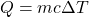# Is Heat a State Function?

#### Engineering

Is heat a state function? The answer is no. Heat is the energy transferred between the system and the surroundings during a process. The amount that transfers does depend on how the process occurs or the state the system takes on. Therefore, heat is not a state function.

Heat is a thermodynamic form of energy contained by any material. The way it changes inside a material depends on the different properties. As heat flows through a system it causes the temperature to fluctuate. In this article, you will learn what heat is, measurements of heat, and examples of state functions.

## What is Heat?

Heat is the way thermal energy passes through or within a system. It measures the energy movement that isn’t caused by mechanical work or potential and kinetic energies. Heat quantifies thermal flow from a higher temperature object to a lower temperature object. Heat and temperature are commonly used interchangeably. However, heat describes the actual movement of thermal energy, and the temperature measures the average energy of motion of the particles of a substance.

As flowing heat spreads out through an entire system, the way heat changes can vary. This is caused by the way heat is defined with respect to the change in internal energy.In the equation above, heat(Q) and the work(W) measure flow into and out of a given point in a system.

Work transfer is reliant on the path it takes to be fully defined, as it equates to force times distance. Heat also is path reliant and prompted by a temperature gradient between two medians. Because of this difference, heat always flows from one point to another.

## Measurements of Heat

One might consider heat having units of °C or °F. However, heat measures energy. This measurement takes the form of Joules, BTUs, amongst other standard measurements.

Temperature is a base unit of measurement systems, whereas heat energy relies on temperature, length, time, and mass. Since heat travels from one point to another in different ways, measuring heat flow proves to be more difficult than measuring temperature.

Calorimetry allows for the accurate measurement of heat energy. This relies mainly on the energy equation but removes the work component to make heat easy to measure. This measurement involves mass(m), specific heat(c), and the change in temperature(ΔT) to define the heat energy.A calorimeter fully determines changes in heat flow throughout the system by holding some values constant. Then, the change in temperature within the system allows for the determination of heat. This allows engineers to better understand how heat flows in different mediums and situations.

Using calorimetry, the measurement of heat becomes relatively simple compared to a full analysis of internal energies. Calorimetry allows engineers to understand the effects of different properties. Examples include how heat flows according to different variations in pressure, temperature, volume, and masses of a given substance. This measurement relies on control volume analysis to fully understand everything that happens within the system.

Consideration of these matters allows for the determination of whether heat is a state function or not.

## Examples of State Functions

A state function relates the properties of a given system to be dependent on the thermodynamic state of the system at that given point. These properties cannot be dependent on the position or path which the state took to reach that point. Common state functions are factors such as pressure, temperature, volume, and entropy.

State functions do not include work and heat. This is because the path that both work and heat take affects the final amount. As such, engineers classify work and heat as path functions. Path functions depend on the route taken. Counter-intuitively, internal energy is a state function even though it depends on a path function.

Internal energy doesn’t rely on the path taken because the difference between points A and B stays the same. Though the energies of heat and work are path-dependent they will always follow the first law of thermodynamics resulting in internal energy being a state function.